## Cubes and Dices

#### Cubes and Dices

1. How many cubes are there in below figure?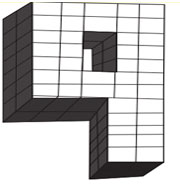1. There are total 42 cubes present in the upper portion.
Then total cubes be 42 × 2 = 84
second method to calculate cubes or blocks.
24 columns with 2 cubes each = 24 × 2 = 48
similarly calculate the number of cubes for others also.

##### Correct Option: A

There are total 42 cubes present in the upper portion.
Then total cubes be 42 × 2 = 84
Second method to find out the number of cubes/blocks.
24 columns with 2 cubes each = 24 × 2 = 48
14 columns with 2 cubes each = 28
4 columns with 2 cubes each = 8
Total cubes = 48 + 28 + 8 = 84

1. How many cubes/blocks are there in below figure?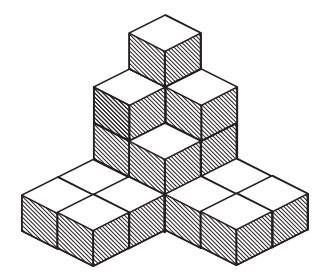1. There are 20 cubes.

##### Correct Option: C

There are 20 cubes.

1. How many cubes/blocks are there in below figure?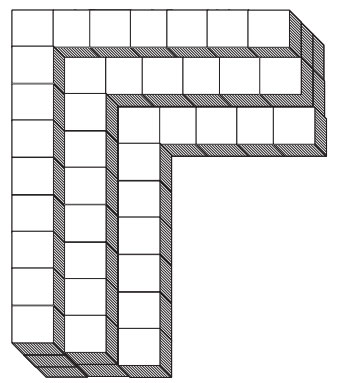1. 15 × 3 + 13 × 2 + 11 × 1

##### Correct Option: D

15 × 3 + 13 × 2 + 11 × 1 = 82 cubes

1. Two positions of a block are shown below (i) and (ii). When 2 is at the bottom which number will be at the top?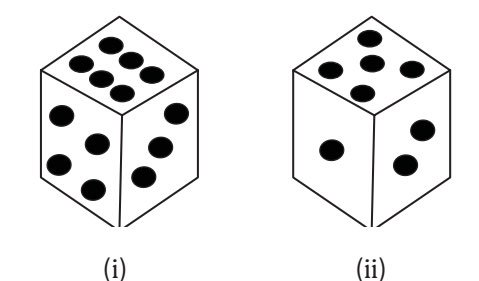1. Number three is common in both the figures we assume the block in figure (ii) to be rotated so that three appears at the same position as in figure (i) and the numbers 5 and 2 move to the faces hidden behind the numbers 4 and 6 respectively.

For the better understanding take a dice and apply the same steps which is mentioned above.

##### Correct Option: C

Number three is common in both the figures we assume the block in figure (ii) to be rotated so that three appears at the same position as in figure (i) and the numbers 5 and 2 move to the faces hidden behind the numbers 4 and 6 respectively. Thus the combined figure will have 3 on right hand side face, 4 on the front face, 6 on the top face, 5 on the rear face and 2 on the bottom face. Clearly when 2 is at the bottom, then 6 is at the top.

1. Two positions of a dice are shown below (i) and (ii). When 3 is at the top which number will be at the bottom?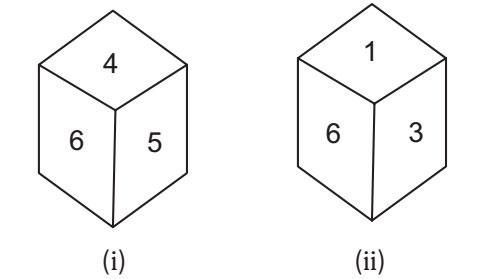1. as number 6 position is same in both the figures. We can assume that the dice to be rotated so that 6 remains on the same face and 1,3,4 and 5 will be arranged ascending order as all are adjacent to 6.
For the better understanding take a dice and apply the same steps which is mentioned above.

##### Correct Option: C

as number 6 position is same in both the figures. We can assume that the dice to be rotated so that 6 remains on the same face and 1,3,4 and 5 will be arranged ascending order as all are adjacent to 6. Hence, when 3 is in top, 5 will be at bottam.